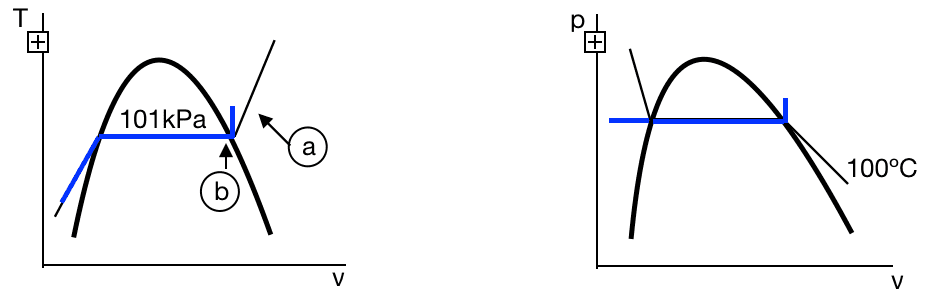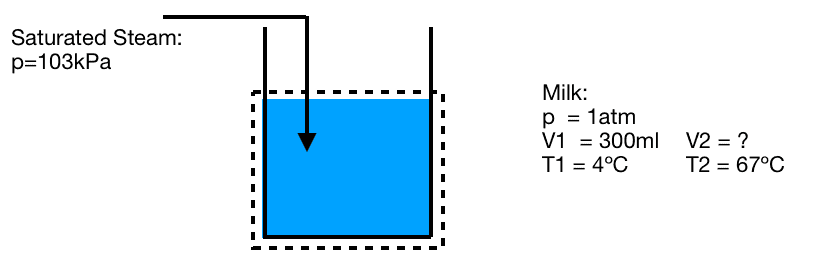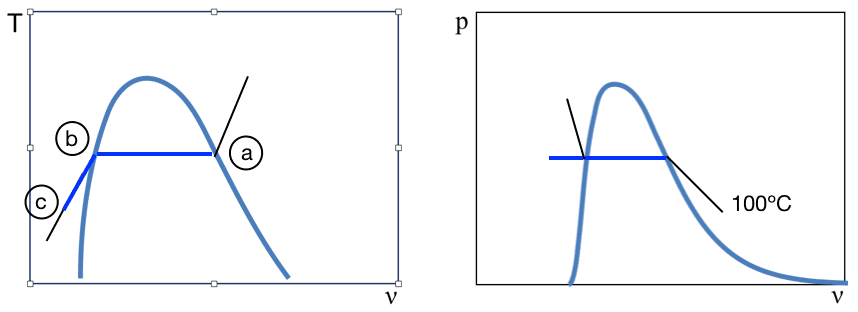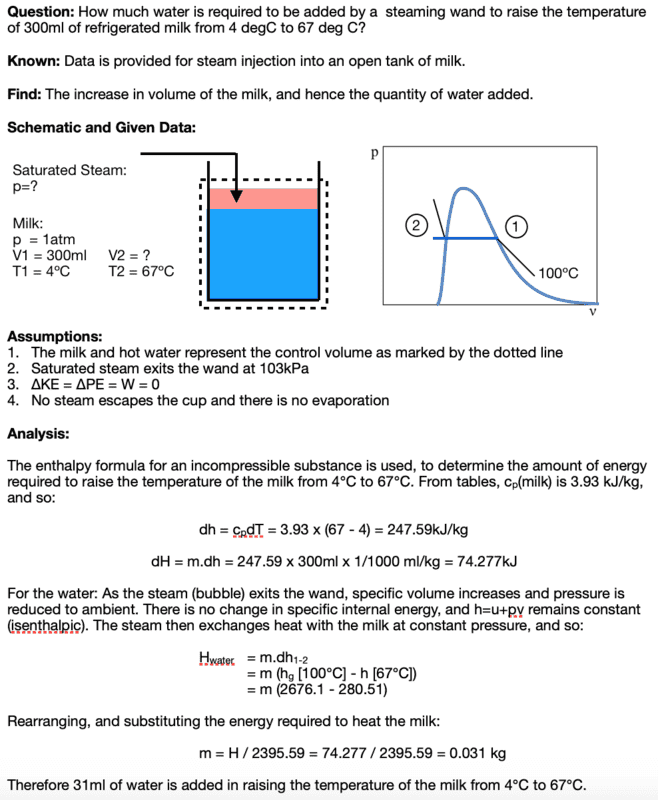# Enthelpy vs Internal Energy in Phase Change

tkyoung75
TL;DR Summary
Request for clarification as to the use of enthalpy vs internal energy data for a phase change from saturated steam to saturated water.
Is there anybody who can advise wether the heat lost / gained during vaporisation, is best calculated from enthalpy, or from internal energy, in the steam tables?

I am trying to establish the increase in the volume of milk when the temperature is raised by adding saturated steam at 1 bar (1 atm, for a coffee). I have been over the problem a couple of times. Generally applying equation of the form Q=dU, where Q is the heat input by the steam and dU change in internal energy of the milk.
In determining Q there is both a condensation component and a cooling component.

For the condensation component, the change in energy comes straight from the steam tables. My book (Moran and Shapiro) gives the enthalpy of vaporisation, however the internal energy of vaporisation must be calculated from the internal energy at saturated liquid and saturated vapour states. Despite the assumptions for a supercooled fluid at constant pressure suggesting that using either enthalpy or internal energy should give the same result, they dont.

As such I am hoping someone might be able to shed some light on why they don't match (i get a 10% difference in the outcome from this component alone), which is the correct one to use. I am presuming that internal energy is the way to go because it is more conservative (condensation) and the enthalpy introduces the pressure component, but on the other hand, enthalpy of vaporisation is the one provided in the book.

Homework Helper
I am not sure how the steam would not be above atmospheric pressure. Is the steam pressure gauge pressure or absolute? In any event, keep in mind that change in enthalpy is equal to Q only if there is no change in P. And ##\Delta U = Q## only if V is constant.

AM

tkyoung75
I am not sure how the steam would not be above atmospheric pressure. Is the steam pressure gauge pressure or absolute? In any event, keep in mind that change in enthalpy is equal to Q only if there is no change in P. And ΔU=QΔU=Q\Delta U = Q only if V is constant.

AM

Thank you Andrew.

The assumed pressure is adjusted to 103kPa, and the discrepancy in h and u resolved using tables.

The following diagrams outline my estimate as to how the steam descends from an elevated pressure and temperature, to the point where it is hot water:
- cooling of superheated steam (103kPa) at constant volume to saturated vapour state
- condensation from saturated vapour to saturated liquid at constant pressure
- cooling of water at constant pressureDoes this look correct?

#### Attachments

Homework Helper
How are you taking into account the decrease in volume of the steam? Keep in mind that:

H = U + PV so ##\Delta H = \Delta U + \Delta(PV) = \Delta U + \int PdV + \int VdP ##

If P is constant then ##\Delta H = Q = \Delta U + \int PdV##

So ##\Delta H## and ##\Delta U## will differ by ##\int PdV##

AM

Last edited:
Mentor
Let's see your detailed analysis of the steam-in-milk problem.

tkyoung75
Question: A steaming wand is used to raise the temperature of 300ml of refrigerated milk from 4 degC to 67 deg C. How much water is added?

Known: Data is provided for steam injection into an open cup of milk.
Find: The increase in volume of the milk, and hence the quantity of water added.
Schematic and Given Data:Assumptions:
1. The milk is a control volume as marked by the dotted line
2. ΔKE = ΔPE = W = 0
3. Milk is an incompressible fluid
4. Density of milk = density of water = density of milk / water mixture
5. Saturated steam exits the wand at 103kPa
Analysis:

To determine the amount of energy required to raise the temperature of the milk, the enthalpy formula for a supercooled fluid at constant temperature is applied. From tables, cp(milk) is 3.93 kJ/kg, and so:

dh = cpdT = 3.93 x (67 - 4) = 247.59kJ/kg

dH = m.dh = 247.59 x 300ml x 1/1000 ml/kg = 74.277kJ

As the steam (bubble) exits the wand, specific volume increases and pressure is reduced to ambient, (Ref: ). There is no change in specific internal energy, and h=u+pv remains constant (isenthalpic). The steam then exchanges heat with the milk at constant pressure in two stages:
- Latent heat exchange as the steam condenses to water
- Isobaric cooling to equilibrium temperaturedhwater = hfg + dhb-c

Applying hfg (100℃) = 2257 kJ/kg, and cp (water) ~ 4.2 kJ/(kgK)

dhwater = 2257 +4.2(100-67) = 2396 kJ/kg = dH/mwater

Therefore, since the water exchanges heat only with the milk,

mwater = dH/dhwater = 74.28 / 2396 = 0.0310 kg

and so the volume of water added is approximately 30ml

Mentor
I don't have time to discuss this now because I have to go to my grandson's hs graduation. But, choosing the milk alone as the control volume is not going to be workable. Try redoing the problem using the total contents of the tank (milk plus added water) as the control volume, rather than just the milk. Assume that none of the steam escapes the tank, and that evaporation is negligible. Try solving it entirely with using the steam tables, rather than heat capacities.

Mentor
What I had in mind was using this as the starting equation for the control volume analysis:
$$m_fu_f-m_iu_i=(m_f-m_i) h_{in} -P_{atm}(m_fv_f-m_iv_i)$$
where m is the mass of liquid in the tank, u is the specific internal energy of the liquid in the tank, ##h_{in}## is the specific enthalpy of the incoming steam, v is the specific volume of the liquid in the tank, f denotes final state, and I denotes initial state.

The only unknown in this equation is ##\Delta m=m_f-m_i##

Last edited:
Mentor
What was the motivation for using 3.93 as the heat capacity? Why is the heat capacity of the mixture taken as a weighted average of water and milk?

tkyoung75
Thank you both for your input.

I have revised the calculation following earlier comments, as below.

What was the motivation for using 3.93 as the heat capacity?

It is the advertised specific heat capacity for milk. It is the only data found for milk (i.e. thermal tables for milk have not been found).

Why is the heat capacity of the mixture taken as a weighted average of water and milk?

Specific heat for water is given in the text for a range of temperatures, and 4.2kJ/kg is selected as accurate to one decimal place for the temperature range in question. This is not used in the revised calculation. I understand that a more accurate result would be obtained by integrating the specific heat as a function of temperature, however for the current exercise I do need to keep it appropriately simple.

In a nutshell, the calculation equates the increase in enthalpy of the milk with the decrease in enthalpy of the water.

Although proper engineering presentation is a must, I feel my solution might still be still a bit fluffy. I like the formula above:
mfuf−miui=(mf−mi)hin−Patm(mfvf−mivi)

however i don't have thermal tables for milk, and its thermal capacity is clearly derated from that for pure water.Mentor
Thank you both for your input.

I have revised the calculation following earlier comments, as below.

It is the advertised specific heat capacity for milk. It is the only data found for milk (i.e. thermal tables for milk have not been found).

Specific heat for water is given in the text for a range of temperatures, and 4.2kJ/kg is selected as accurate to one decimal place for the temperature range in question. This is not used in the revised calculation. I understand that a more accurate result would be obtained by integrating the specific heat as a function of temperature, however for the current exercise I do need to keep it appropriately simple.

In a nutshell, the calculation equates the increase in enthalpy of the milk with the decrease in enthalpy of the water.

Although proper engineering presentation is a must, I feel my solution might still be still a bit fluffy. I like the formula above:

however i don't have thermal tables for milk, and its thermal capacity is clearly derated from that for pure water.

View attachment 244906
Given the assumption that the water and the milk form an ideal solution and that the liquid is incompressible, this looks correct.

•tkyoung75
tkyoung75
Thank you

Mentor
One more thing. I wanted to point out that, even though this problem can be modeled either using an open-system (control volume) description or a closed-system isenthalpic description, if modeled as the latter, it is, strictly speaking, necessary to at least take into consideration that fact that the final enthalpy of the liquid water is not equal to that of saturated liquid water at the final temperature. This is because, in the final state, the liquid water is at a total pressure of 1 atm. rather than at its saturation vapor pressure. So there is an additional term equal to ##v(P_{atm}-p_{sat})## that should be included in the final specific enthalpy of the liquid water. Fortunately, this term has negligible effect on the final results. I should also mention that this term is automatically included in the open-system analysis of the problem.